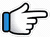# Aur is Dil Mein Kya Rakha Hai – Keyboard Notes## Aur is Dil Mein Kya Rakha Hai Keyboard Notes

For Aur is Dil Mein Kya Rakha Hai Notes as Western or Indian Notations, Click –

Western – CDE FGAB

Indian – srg mpdn

Hindi – सारेग मपधनि

CDE FGAB

सारेग मपधनि

## Aur is Dil Mein Kya Rakha Hai Keyboard Notes

Starting music notes are given
after the notations of the song.

### Part 1

R*~     r*R*    R*~   NDM~
Aur     is        dil      mein

R*r*  R*nn~      NDM
kya__   rakha     hai

Repeat:

R*~   r*R*  R*~ NDM~
Aur   is       dil    mein

R*r*R*nn~ NDM
kya_rakha   hai

M~ DN        DN~ D
Te_ra_ hi    dard
Or
M~ DN       MN~ D

Mgr         gM~     M
chhupa   rakha   hai

Repeat:

M~ DN DN~ D
Tera_ hi dard

Mgr gM~ M
chhupa rakha hai

### Part 2

Notes same as part 1

R*~ r*R*          R*~ NDM
Chee_r_  ke     dekhe

R*r*R*nn~   NDM
dil_ mera     to~~

Repeat:

R*~ r*R*   R*~ NDM
Cheer  ke  dekhe

R*r*R*nn~   NDM
dil_ mera     to~~

M~ DN      DN~ D
Te_ra hi     naam
Or
M~ DN    MN~ D

Mgr     g~ M~   M
likha   rakha     hai

Repeat:

M~ DN     DN~ D
Tera  hi    naam

Mgr    g~ M~ M
likha  rakha   hai

### Music:

Saxophone tone

MR*~~
R*g*r* R*r*R*n~
nR*n   nR*nN

nR*NnR*~
R*g*r*  R*r*R*n
nR*n   nR*nNDM

Guitar Tone (Electric Guitar)

RgMM  NDM
DMg      MgR
RgMM  NDM

RgMM   NDM
D~ Mg   MgR
RgM  NR*

### Part 3

NNn             R*~ R*~ R*~
Pyar_ ke     afsane
Or
N~ n           R*~ R*~ R*~

NNn             R*~ R*~  R*
sune_the    logon         se

D    DN           nn        n
Pya_r _kya    hota    hai,

g*      g*g*      r*g*r*~ R*
yeh   toone    samajaya

Filler:

R*M*~ g* r*g*r*R*

### Part 4

NNn            R*~ R*~    R*~
Mila_ dil    tujh_se      to

NN            nR*~      R*~ R*~
khwaab    dekhe    aise

DD            Nn        nn
Junoon    jaane    kaisa

g*g*       g*~  r*g*     r*~ R*
jawan    dil     pe       chhaya

(Play this first line a little bit faster)

R*    r*R*    R*~ r*R*   R*~ r*R*R*~   r*R*
Dil   mein   aisa          dard_ utha     dil

R*~   g*r*     R*~ nn
ho     gaya    deewana

nn    n~    R* n     nN  NDM
Dil    ho    gaya    dee_wa_na~
Or
nn    nR* R* n     nN  ND  DM
nn    n~  R* n      N~ D~ M~

### Part 5

Notes similar to part 1

R*~ r*R*R*~   NDM~
Deewanon       ne~~

R*r*   R*nn~   NDM
is        duniya    mein

Repeat:

R*~ r*R*R*~   NDM~
Deewanon      ne~~

R*r*   R*nn~    NDM
is        duniya    mein

M~ DN        DN~ D
Dard_ ka    naam

Mgr~     gM~    M
dawa    rakha   hai

Repeat:

M~ DN        DN~ D
Dard_ ka    naam

Mgr~     gM~    M
dawa    rakha   hai

### Repeat Part 1

R*~ r*R* R*~ NDM~, R*r* R*nn~ NDM
Aur is dil mein, kya__ rakha hai

M~ DN DN~ D, Mgr gM~ M
Te_ra__ hi dard, chhupa rakha hai

M~ DN DN~ D, Mgr gM~ M
Te_ra__ hi dard, chhupa rakha hai

### Starting Music Notes:

String Tone:

(Play R* and n 4 times slowly and
then again 4 times fast)

R*~ n~ R*~ n~ R*~ n~ R*~ n~
R*nR*nR*nR*n
R*nNDMgrgM

Guitar Tone (Electric Guitar):

RgMNnN~
(Or RgM n~ N)
MDM    gMM~

RgMNnN~
(Or RgM n~ N)
MDM    gMM~

Immediately next part in string tone (7-8 times G*R*)

G*R*G*R*G*R*G*R*
G*R*G*R*G*R*G*R*
R*

Then start the song notes

Will be Updated

Nigahon mein meri, yeh soorat hai teri
Zindagi yeh meri, amaanat hain teri

Dhadakte seene mein, mohabbat hai teri
Mohabbat hai teri, ibadat hai meri

Tere siwa kuchh yaad nahi hai, Tu hi tu dil mein
Haan tu hi tu dil mein

=

Dil ne yaar ki pooja ki hai
Dil ne yaar ki pooja ki hai
Pyar ka naam khuda rakha hai

## Aur is Dil Mein Kya Rakha Hai Keyboard Notes (from R*)

Part 1

R* ~ r* R* R* ~ NDM~ | R* r* R* nn~ NDM – 2
Aur is dil mein | kya rakha hai – 2

M~ DN DN~ D | Mgr gM~ M – 2
Tera hi dard | chhupa rakha hai – 2

Part 2

R* ~ r* R* R* ~ NDM | R* r* R* nn~ NDM – 2
Cheer ke dekhe | dil mera to~~ – 2

M~ DN DN~ D | Mgr g~ M~ M – 2
Tera hi naam | likha rakha hai – 2

Music:

Saxophone tone

MR* ~~ | R* g* r* R* r* R* n~ | nR* n nR* nN

nR* NnR* ~ | R* g* r* R* r* R* n | nR* n nR* nNDM

Guitar Tone (Electric Guitar)

RgMM NDM | DMg MgR | RgMM NDM

RgMM NDM | D~ Mg MgR | RgM NR*

Part 3

NNn R* ~ R* ~ R* ~ | NNn R* ~ R* ~ R*
Pyar ke afsane | sune the logon se

D DN nn n | g* g* g* r* g* r* ~ R*
Pyar kya hota hai | yeh toone samajaya

Filler:

R* M* ~ g* r* g* r* R*

Part 4

NNn R* ~ R* ~ R* ~ | NN nR* ~ R* ~ R* ~
Mila dil tujhse to | khwaab dekhe aise

DD Nn nn | g* g* g* ~ r* g* r* ~ R*
Junoon jaane kaisa | jawan dil pe chhaya

(Play this first line a little bit faster)

R* r* R* R* ~ r* R* R* ~ r* R* R* ~ r* R* | R* ~ g* r* R* ~ nn
Dil mein aisa dard_ utha dil | ho gaya deewana

nn n~ R* n nN NDM
Dil ho gaya dee_wa_na~

Part 5

R* ~ r* R* R* ~ NDM~ | R* r* R* nn~ NDM – 2
Deewanon ne~~ | is duniya mein – 2

M~ DN DN~ D | Mgr~ gM~ M – 2
Dard_ ka naam | dawa rakha hai – 2

Repeat Part 1

Aur is dil mein, kya rakha hai
Tera hi dard, chhupa rakha hai

Starting Music Notes:

String Tone:

R* ~ n~ R* ~ n~ R* ~ n~ R* ~ n~ | R* nR* nR* nR* n | R* nNDMgrgM

Guitar Tone (Electric Guitar):

RgMNnN~ | MDM gMM~

RgMNnN~ | MDM gMM~

Immediately next part in string tone

G* R* G* R* G* R* G* R* | G* R* G* R* G* R* G* R* | R*

Then start the song notes

Song List

Bhajan List

New Song List